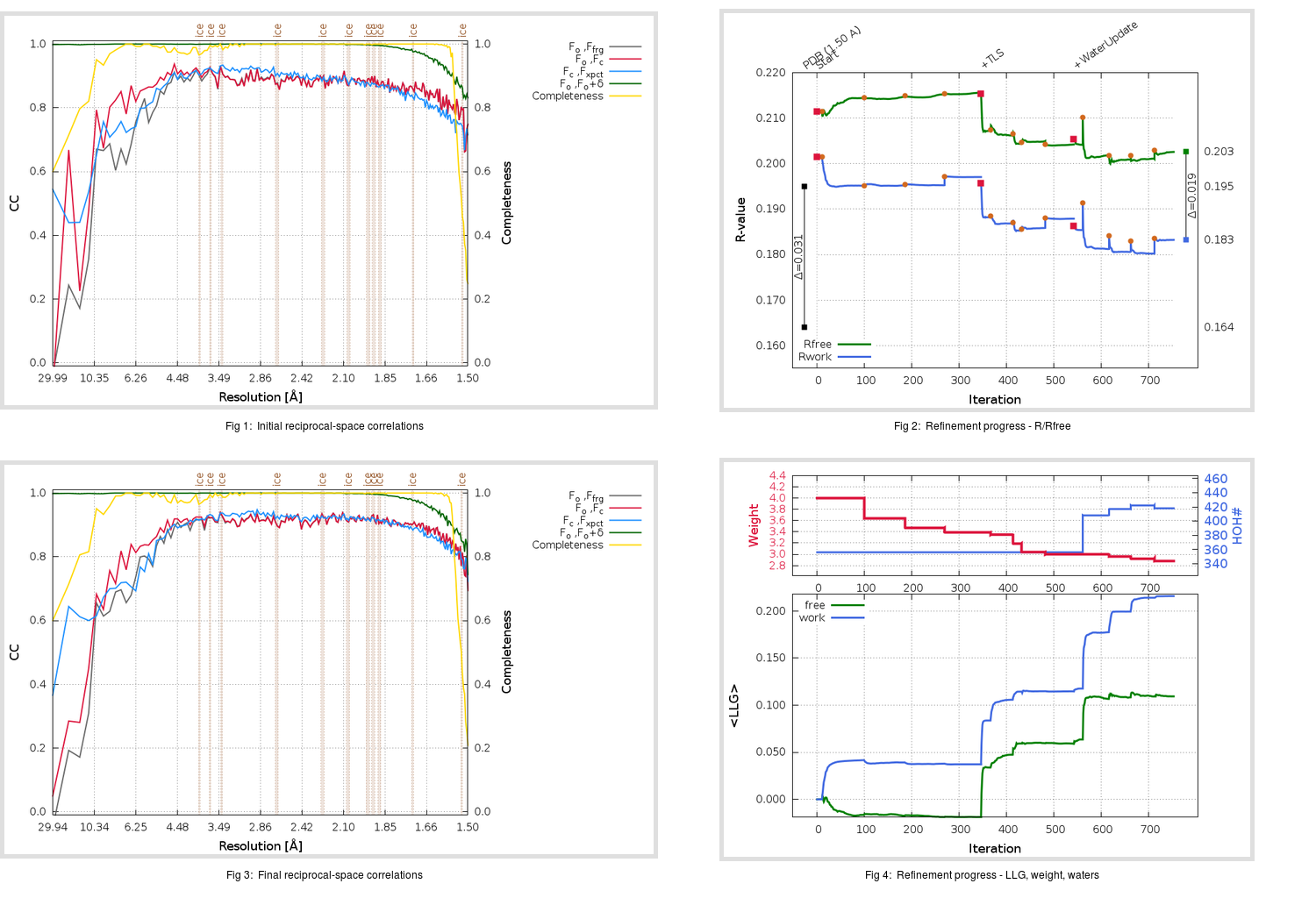Content:

```    Diffraction limits & principal axes of ellipsoid fitted to diffraction cut-off surface:
1.527         1.0000   0.0000   0.0000       a*
1.484         0.0000   1.0000   0.0000       b*
1.493         0.0000   0.0000   1.0000       c*
```

## Deposited

` `
 Date deposited Date data collection Resolution R, Rfree 20170214 20140621 1.50 0.1630 0.1950

Molprobity (CCP4 7.0 version) summary:

```Ramachandran outliers =   0.00 %
favored =  96.37 %
Rotamer outliers      =   1.03 %
C-beta deviations     =     0
Clashscore            =   1.70
RMS(bonds)            =   0.0043
RMS(angles)           =   0.95
MolProbity score      =   1.17
Resolution            =   1.50
R-work                =   0.1630
R-free                =   0.1950
```

```Number of waters      =   356

<B> (all atoms) =   18.00 ( sd =    8.89 ) for       3074 non-hydrogen atoms
<B>   (protein) =   16.10 ( sd =    6.63 ) for       2662 non-hydrogen atoms
<B>     (water) =   31.67 ( sd =   11.43 ) for        356 non-hydrogen atoms
<B>    (others) =   20.59 ( sd =    4.74 ) for         56 non-hydrogen atoms

B min/max       (all non-hydrogen atoms) =    5.67 /   60.87
B min/max   (protein non-hydrogen atoms) =    6.02 /   51.88
B min/max     (water non-hydrogen atoms) =    5.67 /   60.87
B min/max     (other non-hydrogen atoms) =   11.87 /   33.14
```

## BUSTER (re-)refinement

` `

Molprobity (CCP4 7.0 version) summary:

```Ramachandran outliers =   0.00 %
favored =  96.68 %
Rotamer outliers      =   0.69 %
C-beta deviations     =     0
Clashscore            =   1.32
RMS(bonds)            =   0.0111
RMS(angles)           =   1.54
MolProbity score      =   1.07
Resolution            =   1.50
R-work                =   0.1832
R-free                =   0.2026
```

```Number of waters      =   418

<B> (all atoms) =   18.22 ( sd =    8.97 ) for       3136 non-hydrogen atoms
<B>   (protein) =   16.13 ( sd =    6.48 ) for       2662 non-hydrogen atoms
<B>     (water) =   31.16 ( sd =   11.57 ) for        418 non-hydrogen atoms
<B>    (others) =   19.91 ( sd =    4.07 ) for         56 non-hydrogen atoms

B min/max       (all non-hydrogen atoms) =    6.24 /  100.39
B min/max   (protein non-hydrogen atoms) =    6.28 /   49.67
B min/max     (water non-hydrogen atoms) =    6.24 /  100.39
B min/max     (other non-hydrogen atoms) =   13.39 /   28.59
```

Refinement progression:Results:

` `
 File Remark 5X4R_aB_refine.01_03_refine.pdb.gz exact refinement commands are in header 5X4R_aB_refine.01_03_refine.mtz.gz including original deposited data and several re-refinement map coefficients 5X4R_aB_refine.01_03_BUSTER_model.cif.gz including any non-standard compound restraints 5X4R_aB_refine.01_03_BUSTER_refln.cif.gz